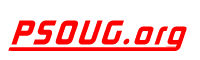Quick Search: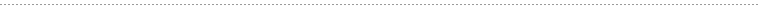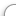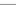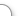CODE Oracle PL/SQL Code Library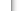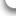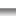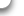JOBS Find Or Post Oracle JobsFORUM Oracle Discussion & ChatPHP Code: Roman2dec and Dec2Roman Jump to: Select a Location PSOUG Home Page Oracle Code Library mySQL Code Library PHP Code Library JavaScript Code Library Oracle Terms & Definitions Oracle Error Codes PSOUG Community Blogs Oracle Jobs Board PSOUG Forum Oracle User Group Directory Free Oracle Magazines Online Learning Center PSOUG Presentations Advanced Code Search News and Events Sponsors Page Submit Code Contact UsSnippet Name: Roman2dec and Dec2Roman

Description: A function that converts to and from Roman numerals.

Comment: (none)

Language: PHP
Highlight Mode: PHP

```<?PHP

FUNCTION dec2roman(\$f)
{
// Return false if either \$f is not a real number, \$f is bigger than 3999 or \$f is lower or equal to 0:
IF(!IS_NUMERIC(\$f) || \$f > 3999 || \$f <= 0) RETURN FALSE;

// Define the roman figures:
\$roman = ARRAY('M' => 1000, 'D' => 500, 'C' => 100, 'L' => 50, 'X' => 10, 'V' => 5, 'I' => 1);

// Calculate the needed roman figures:
FOREACH(\$roman AS \$k => \$v) IF((\$amount[\$k] = FLOOR(\$f / \$v)) > 0) \$f -= \$amount[\$k] * \$v;

// Build the string:
\$return = '';
FOREACH(\$amount AS \$k => \$v)
{
\$return .= \$v <= 3 ? STR_REPEAT(\$k, \$v) : \$k . \$old_k;
\$old_k = \$k;
}

// Replace some spacial cases and return the string:
RETURN STR_REPLACE(ARRAY('VIV','LXL','DCD'), ARRAY('IX','XC','CM'), \$return);
}

// Function to get the decimal value of a roman string:
FUNCTION roman2dec(\$str = '')
{
// Return false if not at least one letter is in the string:
IF(IS_NUMERIC(\$str)) RETURN FALSE;

// Define the roman figures:
\$roman = ARRAY('M' => 1000, 'D' => 500, 'C' => 100, 'L' => 50, 'X' => 10, 'V' => 5, 'I' => 1);

// Convert the string to an array of roman values:
FOR(\$i = 0; \$i < STRLEN(\$str); \$i++) IF(ISSET(\$roman[STRTOUPPER(\$str[\$i])])) \$values[] = \$roman[STRTOUPPER(\$str[\$i])];

// Calculate the sum of that array:
\$sum = 0;
WHILE(\$current = CURRENT(\$values))
{
\$next = NEXT(\$values);
\$next > \$current ? \$sum += \$next - \$current + 0 * NEXT(\$values) : \$sum += \$current;
}

// Return the value:
RETURN \$sum;
}
?>
```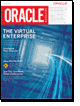FreeOracle MagazineSubscriptionsand Oracle White Papers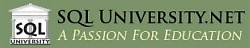SQL University.net courses meet the most demanding needs of the business world for advanced education in a cost-effective manner. SQL University.net courses are available immediately for IT professionals and can be taken without disruption of your workplace schedule or processes.

Compared to traditional travel-based training, SQL University.net saves time and valuable corporate resources, allowing companies to do more with less. That's our mission, and that's what we deliver.